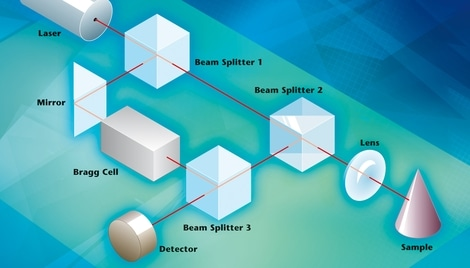# The Basic Principles of Vibrometry

If a wave is reflected by a moving object and detected by a measurement system (as is the case with the LDV), the wave’s measured frequency shift can be expressed as follows:

fD = 2•v/λ

Where, v is the velocity of the object and λ is the wavelength of the emitted wave. An object’s velocity can be determined by measuring the (Doppler-) frequency shift at a known wavelength. In the LDV, this is done by using a laser interferometer.

## Interferometry

The Laser-Doppler vibrometer works on the basis of optical interference, requiring two coherent light beams, with their respective light intensities I1 and I2, to overlap. The resulting intensity is not only the sum of the single intensities, but is modulated in relation to the formula:

Itot = I1 + I2 + 2 √ (I1 I2 cos [2π(r1 - r2)/λ]

with a so-called "interference" term.

This interference term is related to the path length difference between both beams. The overall intensity is four times a single intensity if the path length difference is an integer multiple of the laser wavelength. Correspondingly, if the path length difference between the two beams is half of one wavelength, then the overall intensity is zero.

## Experimental Setup

The following image shows how this physical law is exploited technically in the LDV.At the heart of every Polytec vibrometer system is the laser-Doppler vibrometer – a precision optical transducer used for determining vibration velocity and displacement at a fixed point. The technology is based on the Doppler-effect; sensing the frequency shift of back-scattered light from a moving surface.

A beamsplitter (BS 1) is used to split the beam of a helium neon laser into a reference beam and a measurement beam. Once the measurement beam passes through a second beamsplitter (BS 2), it is focused onto the object under investigation, which reflects it.

The reflected beam is now deflected by BS 2 to merge with the reference beam at the third beam splitter (BS 3), where the merged beam is then directed onto the optical detector.

As the reference beam’s path length is constant over time (with the exception of negligible thermal effects on the interferometer) (r2 = const.), a dark and bright (fringe) pattern typical of interferometry is generated on the detector by a movement of the object of interest (r1 = r(t)).

One complete dark/bright cycle on the detector corresponds to an object displacement of exactly half of the wavelength of the light used. This corresponds to a displacement of 316 nm for the helium neon laser typically used for most vibrometers.

Changing the optical path length over time manifests itself as the Doppler frequency shift of the measurement beam, meaning the modulation frequency of the interferometer pattern measured is directly proportional to the object velocity.

As object movement away from the interferometer creates the same interference pattern (and frequency shift) as object movement toward the interferometer, this experimental setup is not able to determine the direction of object movement.

To perform this task, an acousto-optic modulator (Bragg cell) is introduced into the reference beam to shift the light frequency, typically by 40 MHz, (by comparison, the laser light’s frequency is 4.74 1014 Hz). As a result, a modulation of the frequency of  40 MHz is seen by the detector when the object is at rest.

The modulation frequency is reduced when the object moves toward the interferometer and the detector receives a frequency more than 40 MHz if the object moves away from the vibrometer. As a result, not only the amplitude of movement can be detected, but also the direction of movement can be clearly defined.

## Displacement or Velocity?

In principle, displacement as well as velocity can be directly measured with the LDV. Here, the phase of the electronic carrier signal driving the Bragg Cell is compared with the phase of the detector signal.  Polytec's vibrometers can achieve a resolution of 2 nm using suitable interpolation techniques, and even down to the pm range with digital demodulation techniques

Displacement demodulation is suitable for low frequency measurements and velocity demodulation is better for higher frequencies, because the maximum amplitudes of harmonic vibrations can be described as follows:

v = 2π • f • s

When the frequency of a certain vibration increases, it generates higher velocities at lower displacement amplitudes.This information has been sourced, reviewed and adapted from materials provided by Polytec.

## Citations

• APA

Polytec. (2019, July 05). The Basic Principles of Vibrometry. AZoSensors. Retrieved on October 22, 2020 from https://www.azosensors.com/article.aspx?ArticleID=717.

• MLA

Polytec. "The Basic Principles of Vibrometry". AZoSensors. 22 October 2020. <https://www.azosensors.com/article.aspx?ArticleID=717>.

• Chicago

Polytec. "The Basic Principles of Vibrometry". AZoSensors. https://www.azosensors.com/article.aspx?ArticleID=717. (accessed October 22, 2020).

• Harvard

Polytec. 2019. The Basic Principles of Vibrometry. AZoSensors, viewed 22 October 2020, https://www.azosensors.com/article.aspx?ArticleID=717.

## Tell Us What You Think